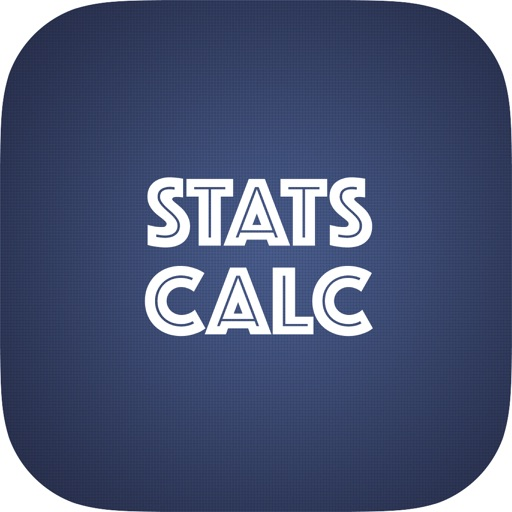Stats Calculator - Statistics Formulas
Removed from the App StoreStats Calculator - Statistics Formulas

Kamal Nasir

1.4.0 for iPhone, iPad
Free
0

0 Ratings

Release Date

2017-05-09

Size

40.9 MB

Screenshots for iPhone

iPhone
More Screenshots for iPad
Stats Calculator - Statistics Formulas Description
This Statistical Calculator performs the following.

1. Arithmetic, Geometric and Harmonic Mean,
2. Arithmetic, Geometric and Harmonic Mean Group Data,
3. Median, Mode and Range,
4. Standard Deviation and Variance,
5. Standard Deviation and Variance Population,
6. Root Mean Square/ Quadratic Mean,
7. Correlation Co-efficient,
8. Simple/Linear Regression,
9. Standard Error Of The Mean,
10. Coefficient Of Variance,
11. Weighted Mean,
12. Bayesian Analysis Calculations,
13. Absolute Percentage Error,
14. Skewness,
15. T Test,
16. F Test,
17. Z Test,
18. Z Score Standard,
19. Skewness Coefficient,
20. Mean Absolute Deviation,
21. Relative Risk Confidence Interval,
22. Empirical Rule,
23. Coefficient Of Determination,
24. Bessel Function,
25. Single Event Probability,
26. Multiple Event Probability,
27. Permutation, Combination,
28. Binomial Distribution,
29. Negative Binomial Distribution,
30. HyperGeometric Distribution,
31. Cumulative HyperGeometric Distribution,
32. Poisson Distribution,
33. Cumulative Poisson Distribution,
34. Uniform Distribution,
35. Multinomial Distribution,
36. Exponential Distribution with Mean Median Standard Deviation Variance,
37. Gamma Distribution,
38. Gamma Formula,
39. Beta Function,
40. Beta Distribution,
41. Normal and Standard Normal Distribution,
42. Normal Population When Alpha Is known,
43. Normal Population When Alpha Is Unknown,
44. Non-Normal Population When Alpha Is known,
45. Confidence Interval For Difference Of Means,
46. Z-Test Normal Population When Alpha Is known,
47. Z-Test Normal Population When Alpha Is Unknown

*Themes let you select a color for the application from different colors.
Price:
Free
Version:
1.4.0
Size:
40.9 MB
Genre:
Education
Release Date:
2017-05-09
Developer:
Kamal Nasir
Language:
English
More
You May Also Like
Developer Apps